CBSE Class 10 Sample Paper for 2020 Boards - Maths Standard

Class 10
Solutions of Sample Papers for Class 10 Boards

## A train covers a distance of 360 km at a uniform speed. Had the speed been 5 km/hour more, it would have taken 48 minutes less for the journey. Find the original speed of the train.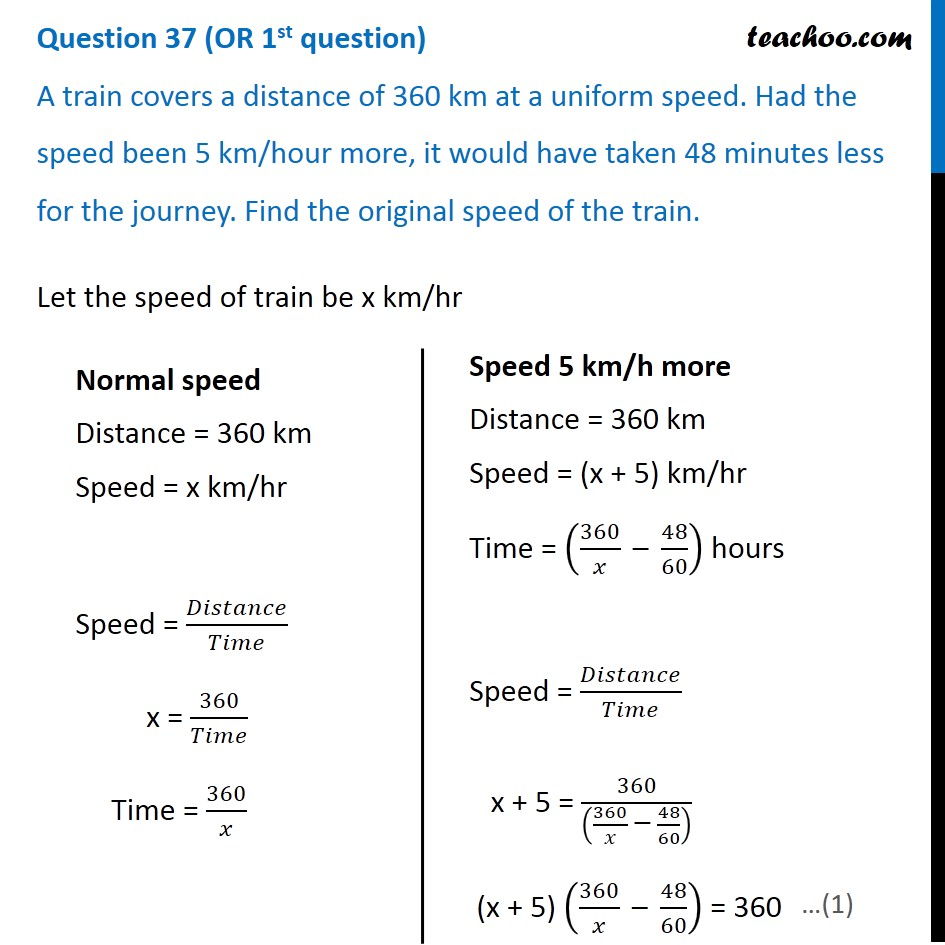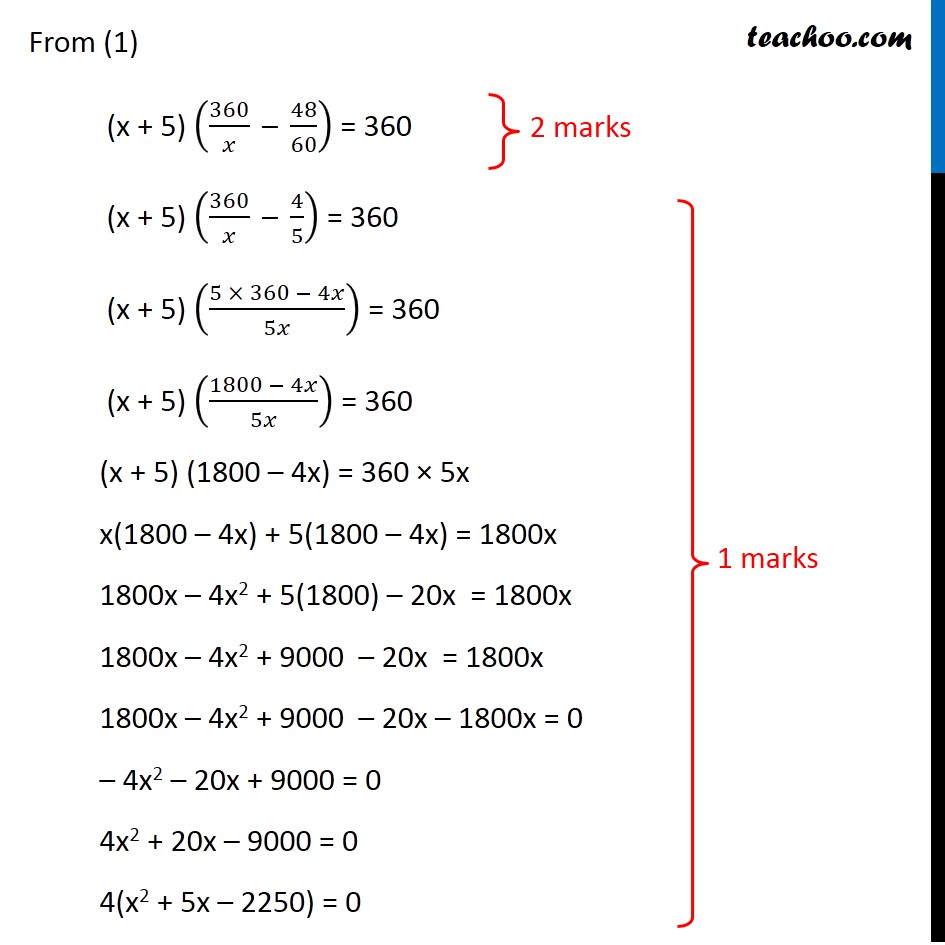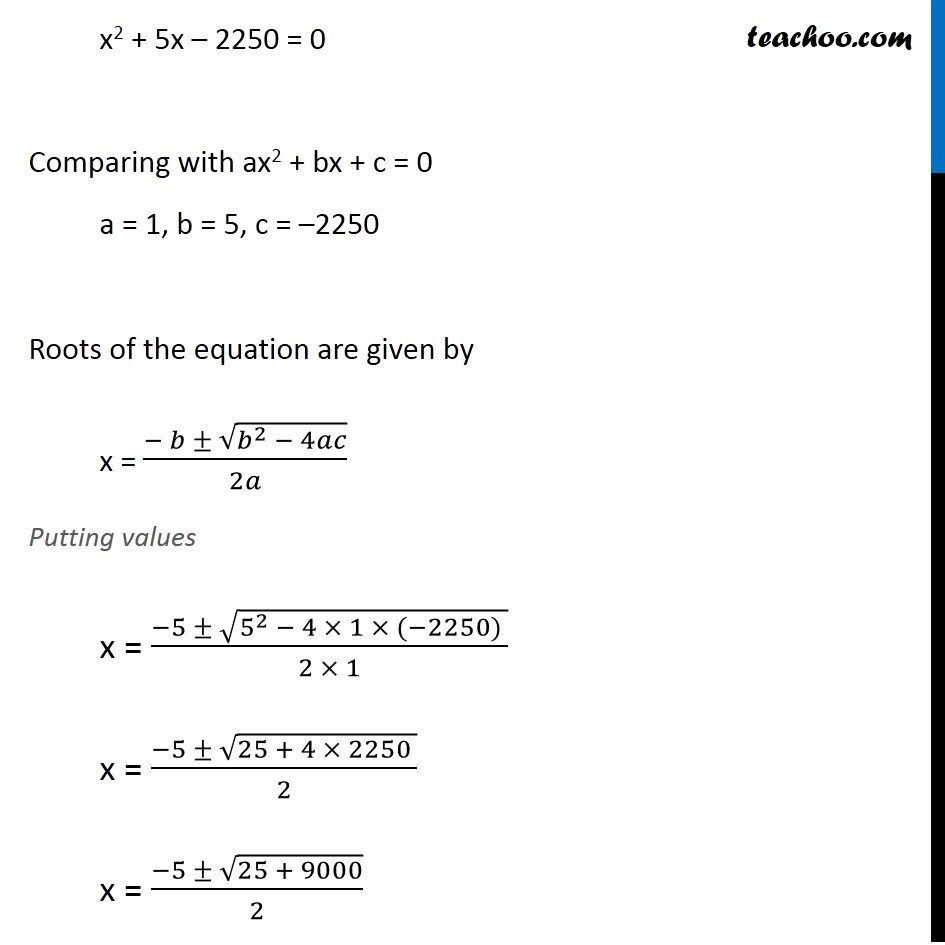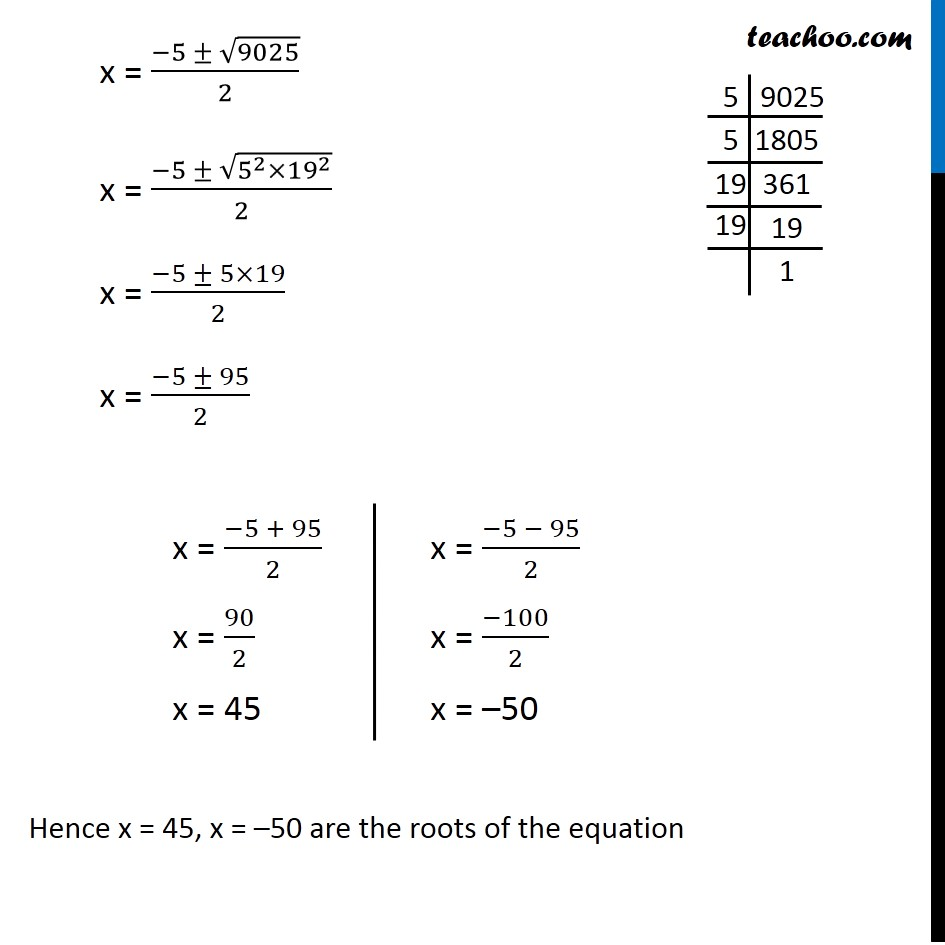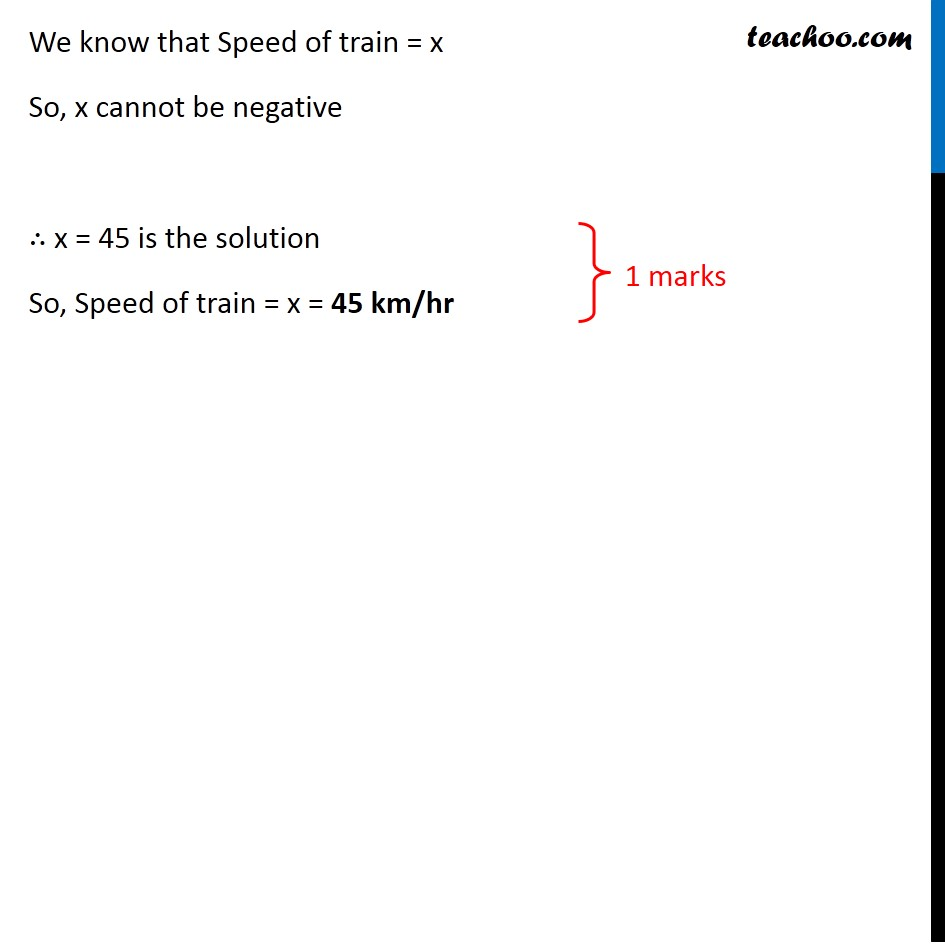Note : This is similar to Ex 4.3, 8 of NCERT – Chapter 4 Class 10

Get live Maths 1-on-1 Classs - Class 6 to 12

### Transcript

Question 37 (OR 1st question) A train covers a distance of 360 km at a uniform speed. Had the speed been 5 km/hour more, it would have taken 48 minutes less for the journey. Find the original speed of the train. Let the speed of train be x km/hr Normal speed Distance = 360 km Speed = x km/hr Speed = 𝐷𝑖𝑠𝑡𝑎𝑛𝑐𝑒/𝑇𝑖𝑚𝑒 x = 360/𝑇𝑖𝑚𝑒 Time = 360/𝑥 Speed 5 km/h more Distance = 360 km Speed = (x + 5) km/hr Time = (360/𝑥 " – " 48/60) hours Speed = 𝐷𝑖𝑠𝑡𝑎𝑛𝑐𝑒/𝑇𝑖𝑚𝑒 x + 5 = 360/((360/𝑥 " – " 48/60) ) (x + 5) (360/𝑥 " – " 48/60) = 360 From (1) (x + 5) (360/𝑥 " – " 48/60) = 360 (x + 5) (360/𝑥 " – " 4/5) = 360 (x + 5) ((5 × 360 − 4𝑥)/5𝑥) = 360 (x + 5) ((1800 − 4𝑥)/5𝑥) = 360 (x + 5) (1800 – 4x) = 360 × 5x x(1800 – 4x) + 5(1800 – 4x) = 1800x 1800x – 4x2 + 5(1800) – 20x = 1800x 1800x – 4x2 + 9000 – 20x = 1800x 1800x – 4x2 + 9000 – 20x – 1800x = 0 – 4x2 – 20x + 9000 = 0 4x2 + 20x – 9000 = 0 4(x2 + 5x – 2250) = 0 x2 + 5x – 2250 = 0 Comparing with ax2 + bx + c = 0 a = 1, b = 5, c = –2250 Roots of the equation are given by x = (− 𝑏 ± √(𝑏^2 − 4𝑎𝑐))/2𝑎 Putting values x = (−5 ± √(5^2 − 4 × 1 × (−2250) ))/(2 × 1) x = (−5 ± √(25 + 4 × 2250 ))/2 x = (−5 ± √(25 + 9000))/2 x = (−5 ± √9025)/2 x = (−5 ± √(5^2×〖19〗^2 ))/2 x = (−5 ± 5×19)/2 x = (−5 ± 95)/2 x = (−5 + 95)/2 x = 90/2 x = 45 x = (−5 − 95)/2 x = (−100)/2 x = –50 Hence x = 45, x = –50 are the roots of the equation We know that Speed of train = x So, x cannot be negative ∴ x = 45 is the solution So, Speed of train = x = 45 km/hr